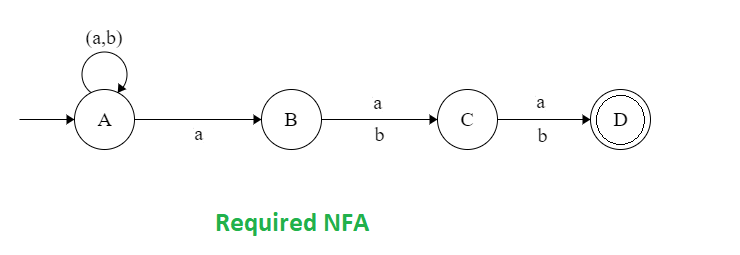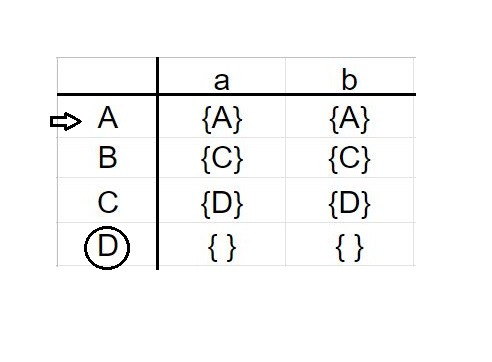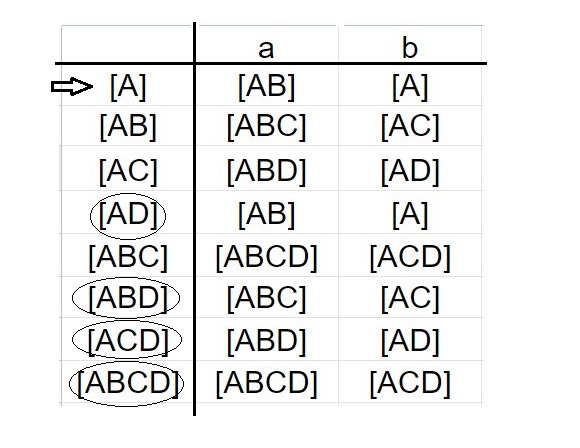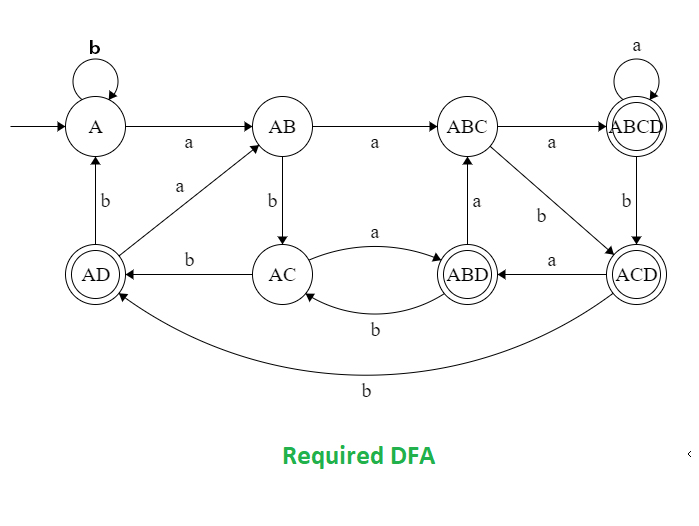Related Articles

# DFA of a string in which 3rd symbol from RHS is ‘a’

• Difficulty Level : Hard
• Last Updated : 06 Sep, 2019

Prerequisite – Finite Automata Introduction, DFA of a string in which 2nd symbol from RHS is ‘a’
Problem – Draw deterministic finite automata (DFA) of the language containing the set of all strings over {a, b} in which 3rd symbol from RHS is ‘a’.

The strings in which 3rd last symbol is “a” are:

Attention reader! Don’t stop learning now.  Practice GATE exam well before the actual exam with the subject-wise and overall quizzes available in GATE Test Series Course.

Learn all GATE CS concepts with Free Live Classes on our youtube channel.

```aaa, aab, aaab, aaaa, aabbaaa, bbbaba etc
```

The DFA which accept the language of such strings is similar the DFA which accept the regular expression:

`L = (a+b)*.a.(a+b).(a+b) `

For example:

```Input: babaa
Output: NOT ACCEPTED

Input: aaabb
Output: ACCEPTED ```

Constructing the DFA of the given problem directly is very complicated. So, here we are going to design the non-deterministic finite automata (NFA) and then convert it to the deterministic finite automata (DFA).

The NFA of the language containing all the strings in which 3rd symbol from the RHS is “a” is:Here, A is the initial state and D is the final state.

Now, we are going to construct the state transition table of the above NFA.After that we will draw the state transition table of DFA using subset configuration on the state transition table of NFA. We will mention all the possible transition for a and b.Now it’s become very easy to draw the DFA with the help of its transition table. In this DFA, we have eight different states A, AB, AC, AD, ABC, ABD, ACD and ABCD, where AD, ABD, ACD and ABCD are the final states and A is the initial state of the DFA.This is our required DFA of the language containing the set of all strings over {a, b} in which 3rd symbol from RHS is ‘a’.

My Personal Notes arrow_drop_up